FEEDBACK

#### Introduction to Matrix Theory

Price: \$12.05 \$8.47 (Save \$3.58)
Only 1 left in stock, order soon.

Author: Wang Zhengsheng;
Language: English
Format: 25.6 x 18.6 x 0.8 cm
Page: 160
Publication Date: 06/2016
ISBN: 9787030453945
Publisher: Science Press
Contents
Chapter 1 Review and Miscellanea: Basic Concepts in Linear Algebra 1
1.1 Matrix Concept and Special Matrices 1
1.2 Matrix Algebra 3
1.3 Eigenvalue and Eigenvector 7
1.4 Reading: Cayley and Sylvester 10
1.5 Self Assessment Exercises 11
Chapter 2 Linear Space and Inner-product Space 13
2.1 Linear Space (Vector Space) 13
2.2 Basis and Dimension of Vector Space 17
2.3 Subspaces 20
2.4 Inner Product Space 21
2.5 Reading: Gram and Schmidt 24
2.6 Self Assessment Exercises 25
Chapter 3 Linear Transformation 27
3.1 Introduction 27
3.2 Linear Transformation with Properties 27
3.3 The Range and Kernel 30
3.4 Matrix Representation of Linear Transformation 32
3.5 Similarity 36
3.6 Reading: Cramer and Gershgorin 38
3.7 Self Assessment Exercises 39
Chapter 4 Jordan Canonical Form 41
4.1 Diagonalizability 41
4.2 Jordan Block Matrix and Jordan Form Matrix 44
4.3 A-matrix and Smith Standard Form 45
4.4 Jordan Canonical Form 49
4.5 Cayley-Hamilton Theorem and Minimal Polynomial 53
4.6 MATLAB Commands for Jordan Canonical Form 55
4.8 Self Assessment Exercises 58
Chapter 5 Matrix Factorization 60
5.1 Introduction 60
5.2 Full Rank Decomposition 60
5.3 LU Factorization 63
5.4 QR Factorization 67
5.5 Schur Decomposition 69
5.6 Singular Value Decomposition(SVD) 71
5.7 An Example of Application: Image Compression 74
5.8 MATLAB Commands for Matrix Factorization 76
5.9 Reading: Householder and Householder Award 78
5.10 Self Assessment Exercises 80
Chapter 6 Hermitian Matrix and Positive Definite Matrix 81
6.1 Hermitian Matrix 81
6.2 Positive Definite Matrix 84
6.4 Self Assessment Exercises 88
Chapter 7 Matrix Norm and Matrix Analysis 89
7.1 Introduction 89
7.2 Vector Norm 89
7.3 Matrix Norm 91
7.4 Matrix Sequence， Series and Function 94
7.5 MATLAB Commands for Norms 98
7.7 Self Assessment Exercises 100
Chapter 8 The Moore-Penrose Generalized Inverse 102
8.1 Introduction 102
8.2 The Moore-Penrose Generalized Inverse 102
8.3 The Solvability of Linear Systems 105
8.4 MATLAB Commands for the Moore-Penrose Generalized Inverse Matrix 107
8.5 Reading: Moore and Penrose 108
8.6 Self Assessment Exercises 109
Chapter 9 An Introduction to MATLAB 110
9.1 A Glance of MATLAB 110
9.2 Starting Up 111
9.3 MATLAB as A Calculator 112
9.4 Plotting 119
9.5 Programming 122
9.6 Commonly Used Commands Summary 127
9.7 Reading: Golub and Moler 130
Bibliography 149
##### Sample Pages Preview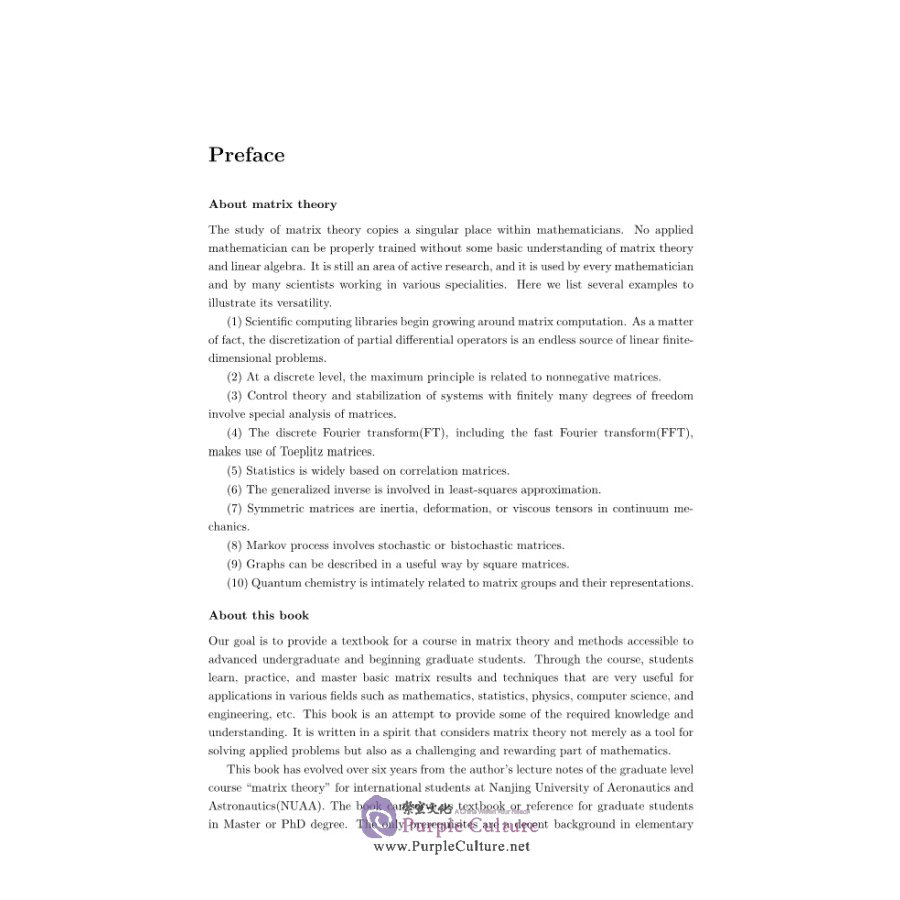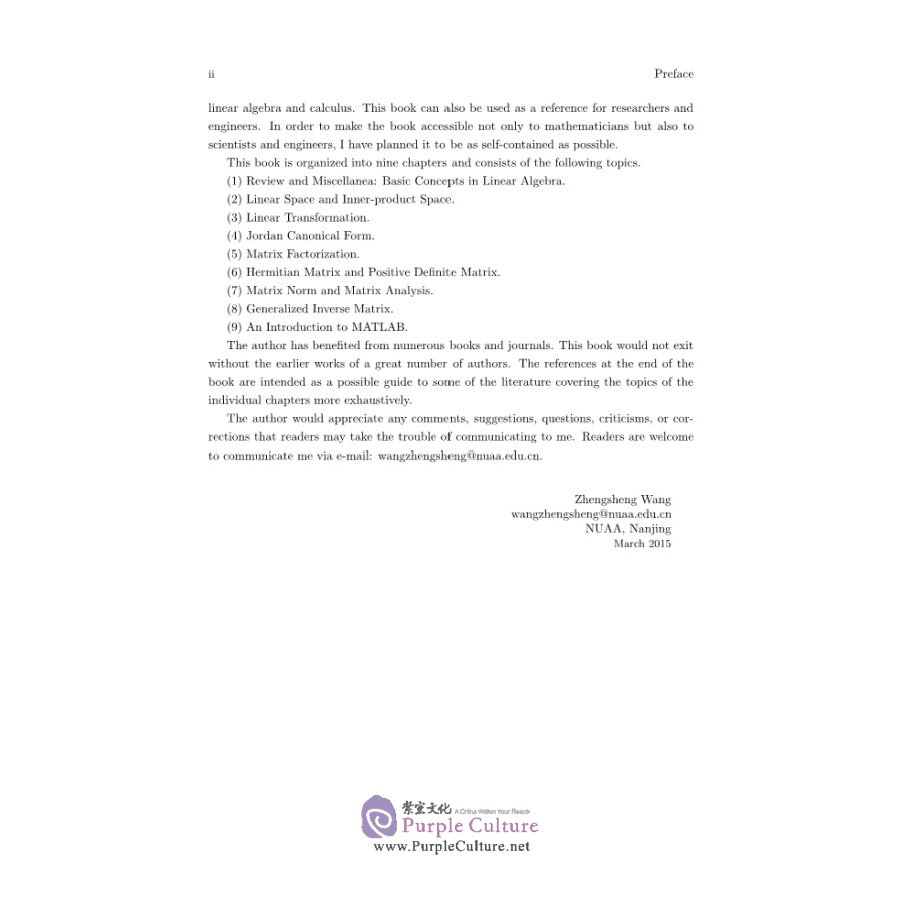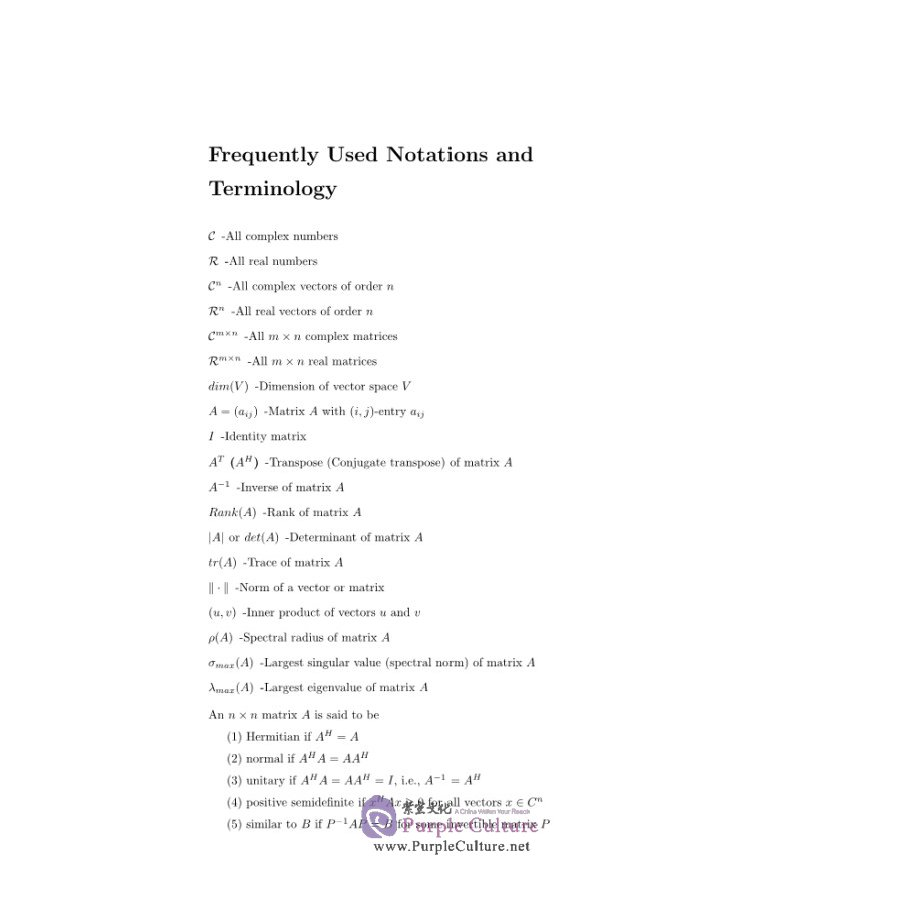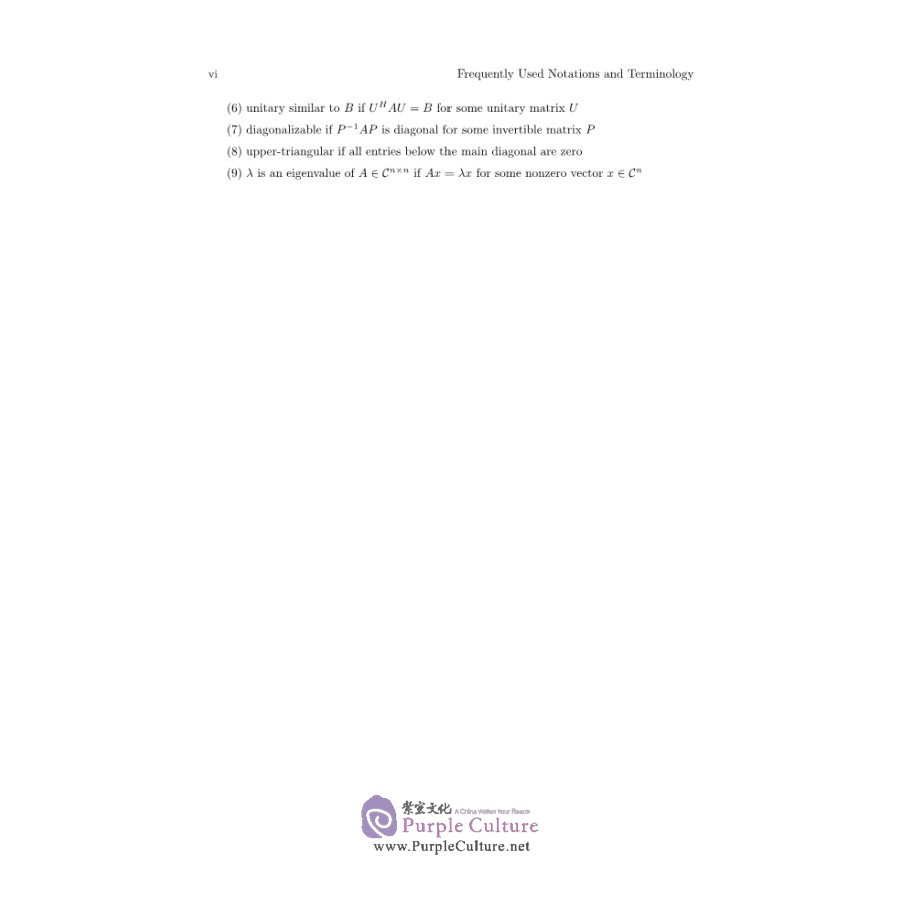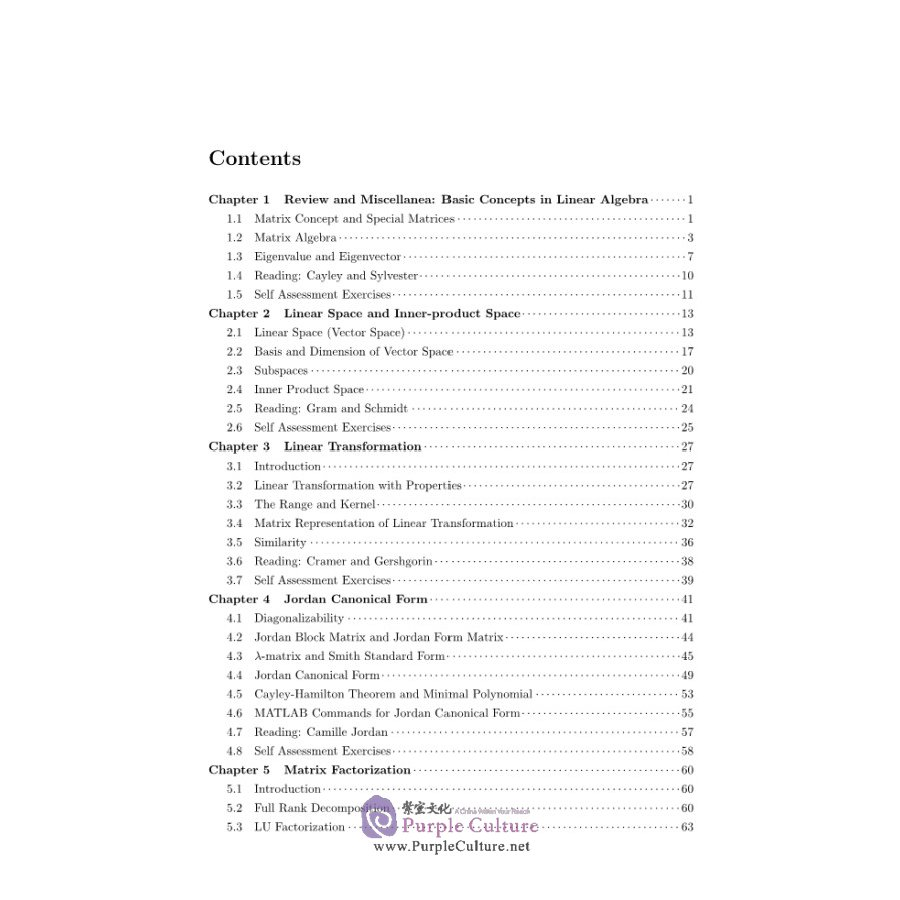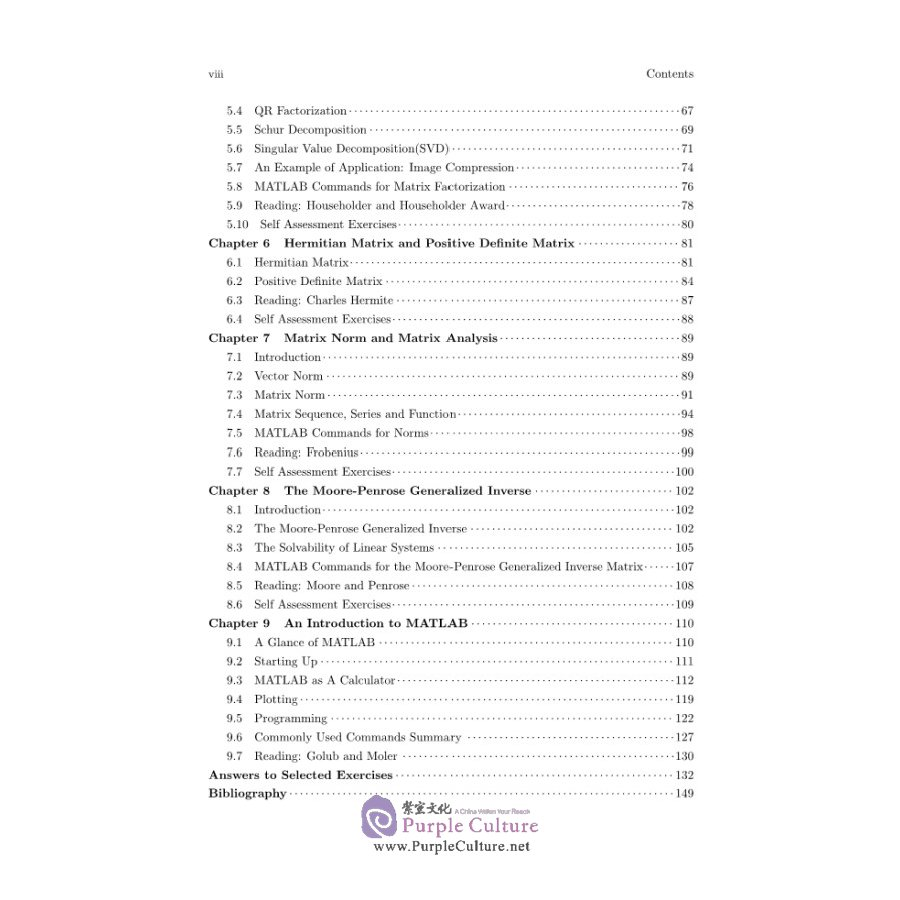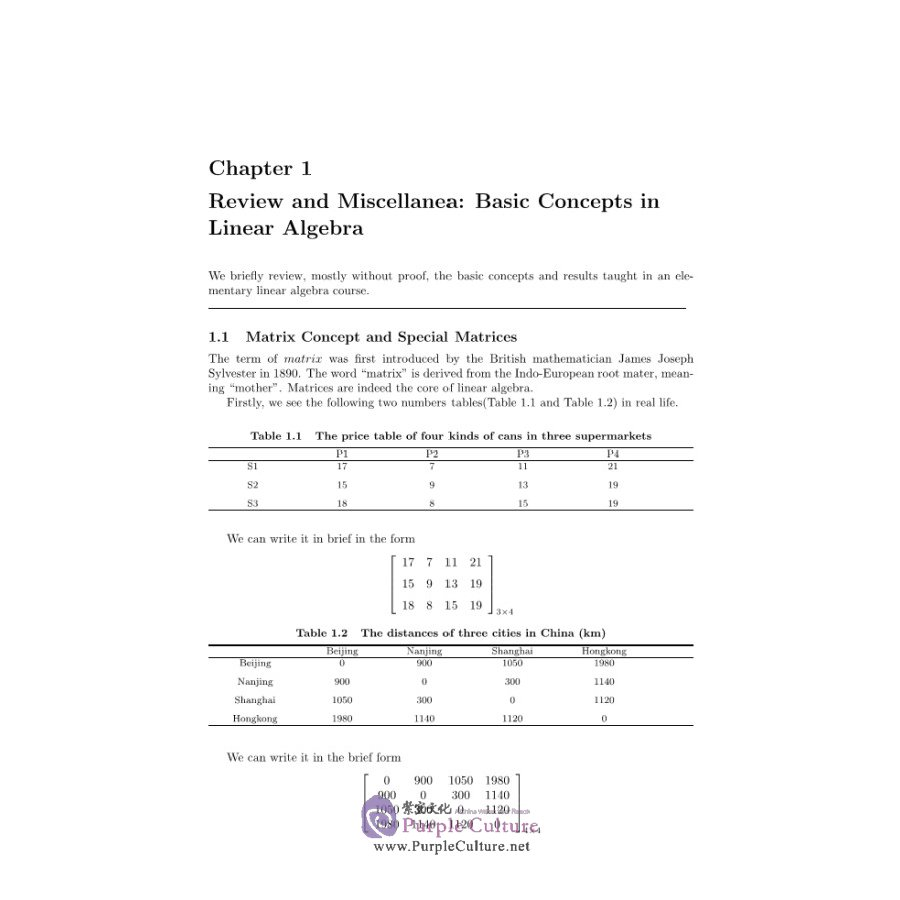\$8.47